###### NIR data correlograms with Seaborn in Python
01/03/2019Detecting outliers in a set of data is always a tricky business. How do we know a data point is an outlier? How do we make sure we are detecting and discarding only true outliers and not cherry-picking from the data? Well, all of these are rhetorical questions, and we can’t obviously give a general answer to them. We can however work out a few good methods to help us make judgements.

Today we are going to discuss one of these good methods, namely the Mahalanobis distance for outlier detection. The aficionados of this blog may remember that we already discussed a (fairly involved) method to detect outliers using Partial Least Squares. If you want to refresh your memory click the button below.

What we are going to work out today is instead a (simpler) method, very useful for classification problems. The PLS-based method is great when you have the primary reference values associated with your spectra (the “labels”), but can’t be used for unlabelled data.

Conversely, Principal Components Analysis (PCA) can be used also on unlabelled data – it’s very useful for classification problems or exploratory analysis. Therefore we can use PCA as a stepping stone for outliers detection in classification. For a couple of our previous posts on PCA check out the links below.
[/one]

# PCA score plots of NIR data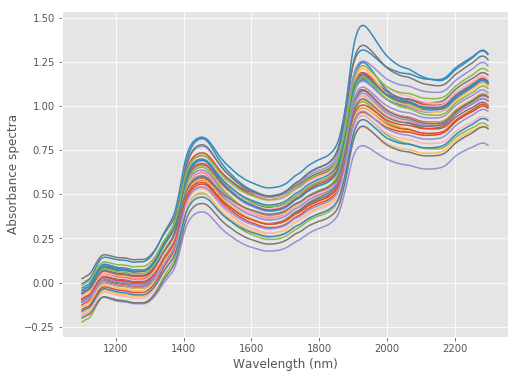For this tutorial, we are going to use NIR reflectance data of fresh plums taken with a Brimrose Luminar 5030 spectrometer. Data are acquired from 1100 to 2300 nm with step of 2 nm. Here’s how the data look like:

And here’s the code required to load and plot the data.

Now it’s time to run a PCA decomposition of these data and produce a score plot with the first two principal components.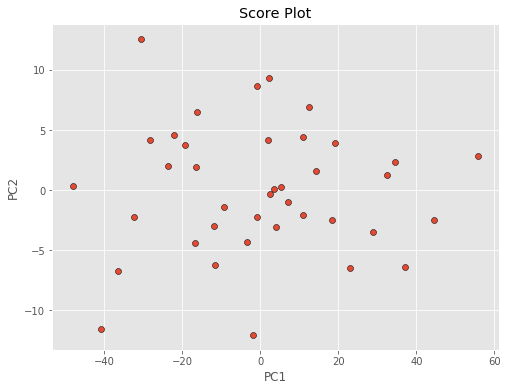So far so good. We are now going to use the score plot to detect outliers. More precisely, we are going to define a specific metric that will enable to identify potential outliers objectively. This metric is the Mahalanobis distance.

But before I can tell you all about the Mahalanobis distance, I need to tell you about another, more conventional distance metric, called the Euclidean distance.

# Euclidean distance for score plots

The Euclidean distance is what normal people call “distance”. That is the conventional geometrical distance between two points. Consider the score plot above. Pick any two points. The distance between the two (according to the score plot units) is the Euclidean distance.

I know, that’s fairly obvious… The reason why we bother talking about Euclidean distance in the first place (and incidentally the reason why you should keep reading) is that things get more complicated when we want to define the distance between a point and a distribution of points. In the good books, this is called “multivariate” distance.

This is the whole business about outliers detection. We define an outlier in a set of data as a point which is “far” (according to our distance metric) from the average of that set. Again, look at the score plot above. I bet you can approximately pinpoint the location of the average (or centroid) of the cloud of points, and therefore easily identify the points which are closer to the centre and those sitting closer to the edges.

This concept can be made mathematically precise. The Euclidean distance between a point and a distribution is given by $$z = (x – \mu)/\sigma$$ where $$x$$ is the point in question, $$\mu$$ is the mean and $$\sigma$$ the standard deviation of the underlying distribution. $$\sigma$$ is there to guarantee that the distance measure is not skewed by the units (or the range) of the principal components.

If you look closely at the axes of the score plot above, you’ll notice that PC1 ranges roughly between -40 and 60, while PC2 between (roughly) -12 and 12. If we drew the score plot using the correct aspect ratio, the cloud of point would squash to an ellipsoidal shape. In fact let’s redraw the score plot just so.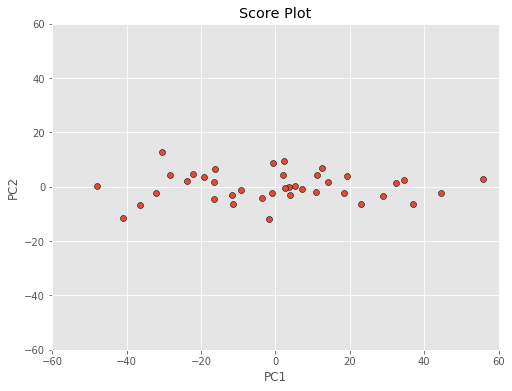By normalising the measure by the standard deviation, we effectively normalise the range of the different principal components, so that the standard deviation on both axis becomes equal to 1.

Finally, to add another layer of complication, we can generalise the Euclidean distance to more than two principal components. Even if we can’t visualise it, we can conceive of a score plot in, say, 5 dimensions. If for instance we decide to use 5 principal components we can calculate the Euclidean distance with this neat piece of code

This code calculates the Euclidean distance of all points at once. Better still, we can use the Euclidean distance (in 5D!) to colour code the score plot. Take a look.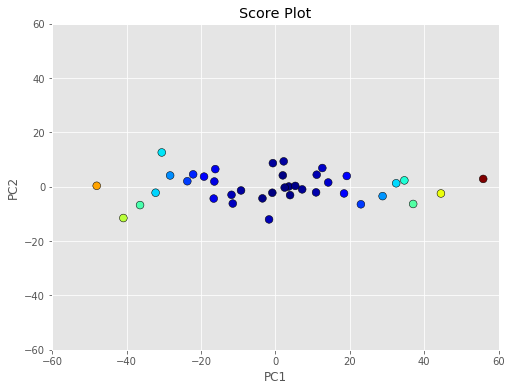As you can see, the points towards the edges of along PC1 tends to have larger distances. More or less as expected.

There is however a problem lurking in the dark. Here’s where we need the Mahalanobis distance to sort it out.

# Mahalanobis distance for score plots

In fact, there are in general two problems with the Euclidean distance. The first problem does not apply to here, but it might exist in general, so I better mention it. If there happened to be a correlation between the axes (for instance if the score plot ellipsoid was tilted at an angle) that would affect the calculation of the Euclidean distance. In practice Euclidean distance puts more weight than it should on correlated variables.

The reason for that is that can be easily explained with an example. Suppose we had two points that were exactly overlapping (that’s complete correlation). Clearly adding the second point doesn’t add any information to the problem. The Euclidean distance however has no way of knowing those two points are identical, and will essentially count the same data twice. This would put excessive weight on the points in question. The problem is somewhat reduced when there is partial correlation, nevertheless it is something to be avoided in general.

The major problem with the approach above is in the calculation of mean and standard deviation. If we really had outliers in our data, they would definitely skew the calculation of mean and standard deviation. Remember, the outliers are points that do not belong to the distribution. They corresponds to bad measurements (or bad samples) which are not representative of the real distribution. This is why we want to discard them!

However, in a classic chicken and egg situation, we can’t know they are outliers until we calculate the stats of the distribution, except the stats of the distribution are skewed by outliers!

The way out of this mess is the Mahalanobis distance.

Its definition is very similar to the Euclidean distance, except each element of the summation is weighted by the corresponding element of the covariance matrix of the data. The details of the calculation are not really needed, as scikit-learn has a handy function to calculate the Mahalanobis distance based on a robust estimation of the covariance matrix. To learn more about the robust covariance estimation, take a look at this example. The robust estimation takes care of the potential presence of outliers and it goes like this.

Again, we’ve done the calculation in 5D, using the first five principal components. Now we can colour code the score plot using the Mahalanobis distance instead.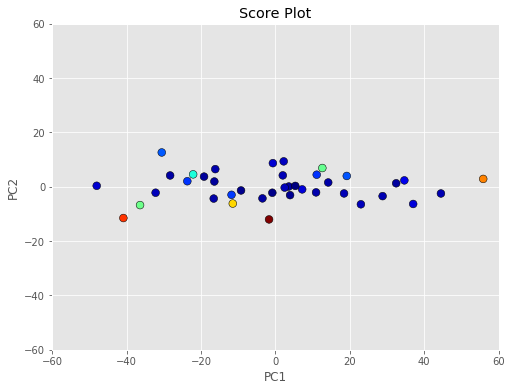There is some notable difference between this and the previous case. Some of the points towards the centre of the distribution, seemingly beyond suspicion, have indeed a large value of the Mahalanobis distance. This doesn’t necessarily mean they are outliers, perhaps some of the higher principal components are way off for those points. In any case this procedure would flag potential outliers for further investigation.

Wrapping up, here’s a fairly unbiased way to go about detecting outliers in unlabelled data. Hope you found it useful. If you’d like to follow along and need the data just give us a shout.

Thanks for reading and until next time!##### Daniel Pelliccia
Physicist and an entrepreneur. Founder and Managing Director at Instruments & Data Tools, specialising in optical design and analytical instrumentation. Founder at Rubens Technologies, the intelligence system for the fresh fruit export industry.